UIHistories Project: A History of the University of Illinois by Kalev Leetaru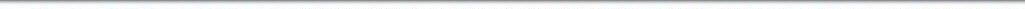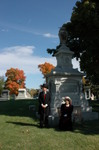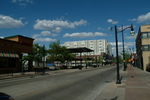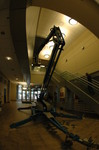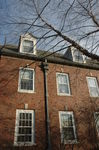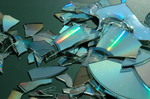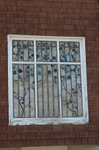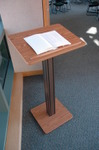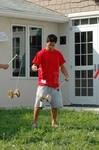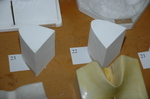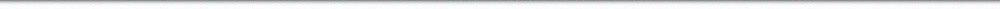N A V I G A T I O N D I G I T A L L I B R A R Y

## Repository: UIHistories Project: Book - Overview of University of Illinois for Chicago Alumni (1926) [PAGE 25]

Caption: Book - Overview of University of Illinois for Chicago Alumni (1926)
This is a reduced-resolution page image for fast online browsing.

< Previous Page [Displaying Page 25 of 63] Next Page >
[VIEW ALL PAGE THUMBNAILS]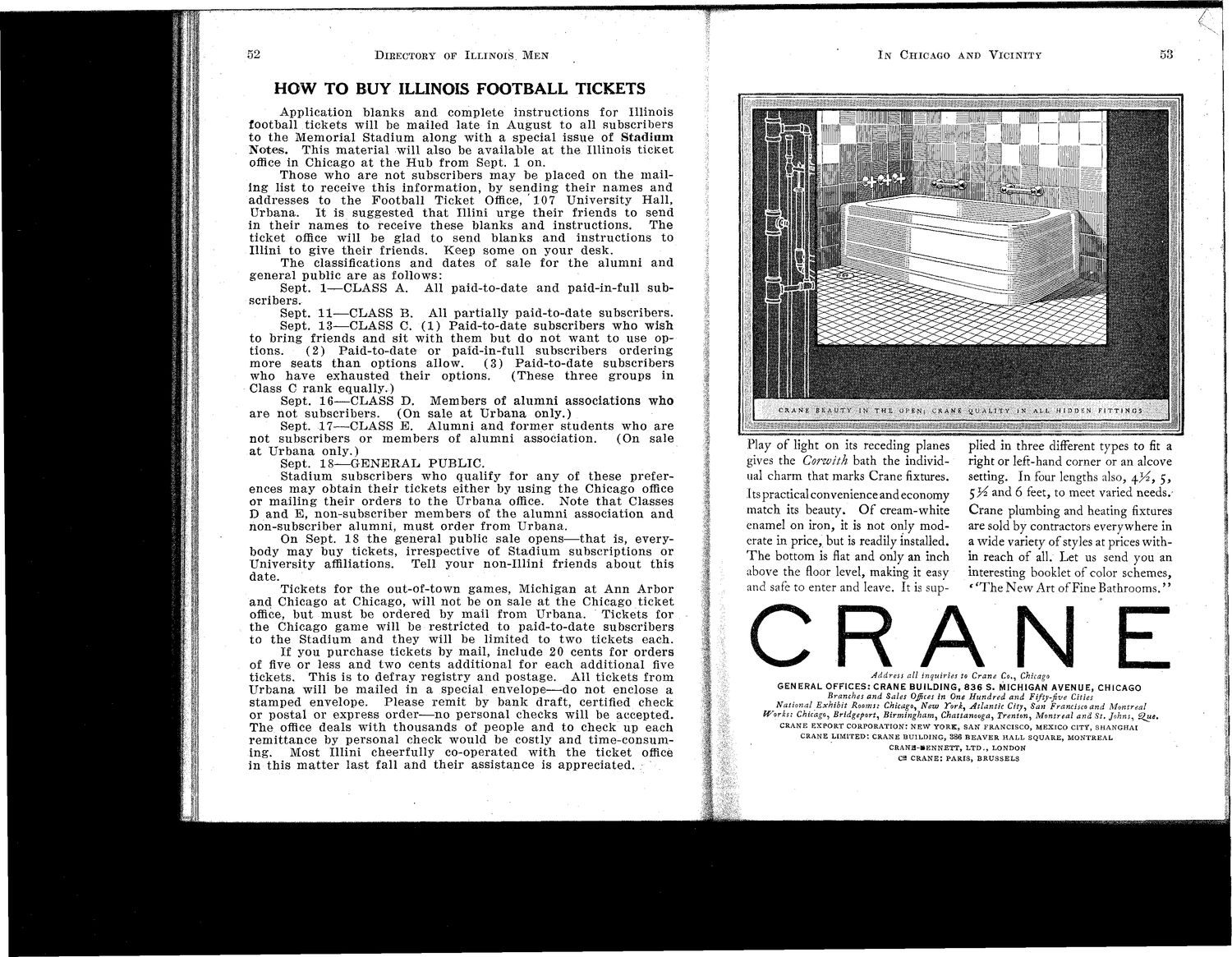### EXTRACTED TEXT FROM PAGE:

52

DlKECTOEY OF ILLINOIS M E N

IN

CHICAGO AND V I C I N I T Y

53

HOW TO BUY ILLINOIS FOOTBALL TICKETS

A p p l i c a t i o n b l a n k s and. c o m p l e t e i n s t r u c t i o n s for Illinois football t i c k e t s will be m a i l e d l a t e in A u g u s t to all s u b s c r i b e r s to t h e M e m o r i a l S t a d i u m a l o n g w i t h a special issue of Stadium N o t e s . T h i s m a t e r i a l will also be a v a i l a b l e a t t h e Illinois t i c k e t office in Chicago a t t h e H u b from Sept. 1 on. T h o s e w h o a r e n o t s u b s c r i b e r s m a y be placed on t h e m a i l i n g list t o receive t h i s i n f o r m a t i o n , by s e n d i n g t h e i r n a m e s a n d a d d r e s s e s to t h e F o o t b a l l Ticket Office, 107 U n i v e r s i t y H a l l , U r b a n a . I t is s u g g e s t e d t h a t Illini u r g e t h e i r friends to send in t h e i r n a m e s t a receive t h e s e b l a n k s a n d i n s t r u c t i o n s . The t i c k e t office will be glad to send b l a n k s a n d i n s t r u c t i o n s to Illini to give t h e i r friends. K e e p s o m e on y o u r d e s k . T h e classifications a n d d a t e s of sale for t h e a l u m n i a n d g e n e r a l public a r e as follows: Sept. 1—CLASS A. All p a i d - t o - d a t e a n d paid-in-full s u b scribers. Sept. 1 1 — C L A S S B. All p a r t i a l l y p a i d - t o - d a t e s u b s c r i b e r s . Sept. 1 3 — C L A S S C. ( 1 ) P a i d - t o - d a t e s u b s c r i b e r s w h o wish to b r i n g friends a n d sit w i t h t h e m b u t do n o t w a n t to use options. ( 2 ) P a i d - t o - d a t e or paid-in-full s u b s c r i b e r s o r d e r i n g m o r e s e a t s t h a n options allow. (3) Paid-to-date subscribers who h a v e e x h a u s t e d t h e i r options. ( T h e s e t h r e e g r o u p s in Class C r a n k e q u a l l y . ) Sept. 1 6 — C L A S S D. M e m b e r s of a l u m n i a s s o c i a t i o n s w h o are not subscribers. (On s a l e a t U r b a n a only.) Sept. 1 7 — C L A S S E. A l u m n i a n d f o r m e r s t u d e n t s w h o a r e n o t s u b s c r i b e r s or m e m b e r s of a l u m n i a s s o c i a t i o n . (On sale a t U r b a n a only.) Sept. 1 8 — G E N E R A L P U B L I C . S t a d i u m s u b s c r i b e r s w h o qualify for a n y of t h e s e p r e f e r ences m a y o b t a i n t h e i r t i c k e t s e i t h e r by u s i n g t h e Chicago office or m a i l i n g t h e i r o r d e r s to t h e U r b a n a office. Note t h a t Classes D a n d E, n o n - s u b s c r i b e r m e m b e r s of t h e a l u m n i association a n d n o n - s u b s c r i b e r a l u m n i , m u s t o r d e r from U r b a n a . On Sept. 18 t h e g e n e r a l public sale o p e n s — t h a t is, everybody m a y buy t i c k e t s , i r r e s p e c t i v e of S t a d i u m s u b s c r i p t i o n s or U n i v e r s i t y affiliations. Tell y o u r n o n - I l l i n i friends a b o u t t h i s date. T i c k e t s for t h e out-of-town g a m e s , Michigan a t A n n A r b o r a n d Chicago a t Chicago, will not be on sale a t t h e Chicago t i c k e t office, b u t m u s t be o r d e r e d by m a i l from U r b a n a . T i c k e t s for t h e Chicago g a m e will be r e s t r i c t e d to p a i d - t o - d a t e s u b s c r i b e r s to t h e S t a d i u m a n d t h e y will be l i m i t e d to t w o t i c k e t s each. If you p u r c h a s e t i c k e t s by m a i l , i n c l u d e 20 c e n t s for o r d e r s of five or less a n d two cents a d d i t i o n a l for each a d d i t i o n a l five t i c k e t s . T h i s is to d e f r a y r e g i s t r y a n d p o s t a g e . All t i c k e t s from U r b a n a will be m a i l e d in a special e n v e l o p e — d o n o t enclose a s t a m p e d envelope. P l e a s e r e m i t by b a n k draft, certified check or p o s t a l or e x p r e s s o r d e r — n o p e r s o n a l c h e c k s will be a c c e p t e d . T h e office d e a l s w i t h t h o u s a n d s of people a n d to check up each r e m i t t a n c e by p e r s o n a l check would be costly a n d t i m e - c o n s u m ing. Most Illini cheerfully co-operated w i t h t h e t i c k e t office in t h i s m a t t e r l a s t fall a n d t h e i r a s s i s t a n c e is a p p r e c i a t e d .

Flay of light on its receding planes gives the Corwith bath the individual charm that marks Crane fixtures. I ts practical convenience and economy match its beauty. O f cream-white enamel on iron, it is not only moderate in price, but is readily installed. T h e bottom is flat and only an inch above the floor level, making it easy and safe to enter and leave. It is sup-

plied in three different types to fit a right or left-hand corner or an alcove setting. In four lengths also, ^.}4, 5, 5>^ and 6 feet, to meet varied needs.Crane plumbing and heating fixtures are sold by contractors everywhere in a wide variety of styles at prices within reach of all. Let us send you an interesting booklet of color schemes, f ' T h e N e w Art of Fine Bathrooms.''

CRAN E

Address all inquiries to Crane Co., Chicago GENERAL OFFICES: CRANE BUILDING, 8 3 6 S. MICHIGAN AVENUE, CHICAGO Branches and Sales Offices in One Hundred and Fifty-five Cities National Exhibit Rooms: Chicago, New York, Atlantic City, San Francisco and Montreal Works: Chicago, Bridgeport, Birmingham, Chattanooga, Trenton, Montreal and St. Johns, ^ue.

CRANE EXPORT CORPORATION: NEW YORK, SAN FRANCISCO, MEXICO CITY, SHANGHAI CRANE LIMITED: CRANE BUILDING, 386 BEAVER HALL SQUARE, MONTREAL CRANB-*ENNETT, L T D . , LONDON C2 CRANE: PARIS, BRUSSELS